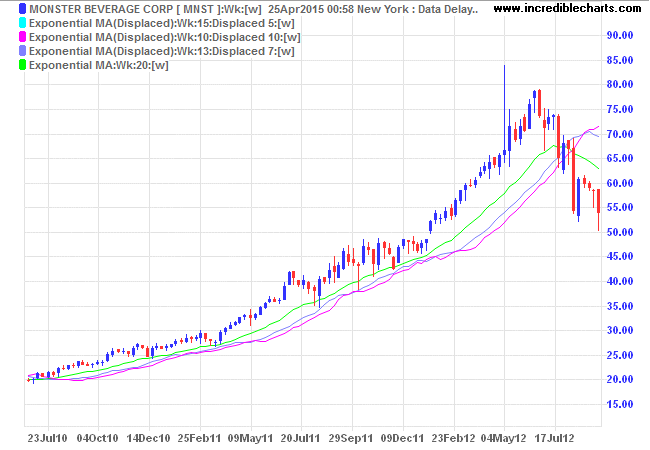# Displaced Moving Average (DMA)

Displaced Moving Averages are useful for trend-following purposes, reducing the number of whipsaws compared to an equivalent Exponential or Simple Moving Average.

Displaced Moving Average generates signals when price crosses the moving average:

• Go long when price crosses to above the Displaced Moving Average from below.
• Go short when price crosses to below the Displaced Moving Average from above.

### Example

Monster Beverage Inc. (MNST) is plotted with a 20-week Simple Moving Average to follow the 2010 to 2012 primary up-trend. The trend is exited just below \$68 in July 2012, but there are 7 whipsaws (exit and re-entry) over the period.Displacing the moving average allows us to minimize the number of whipsaws. Three Displaced Moving Averages are displayed:

• 15-Week displaced by 5 weeks;
• 13-Week displaced by 7 weeks; and
• 10-Week displaced by 10 weeks.

The sum of the Moving Average Period and the Displacement Period in each case adds up to the same 20 weeks as used in the original trend-following moving average. Increasing the Displacement Period reduces responsiveness (or slows the Displaced MA) more than the compensating decrease in the MA Time Period.The trade-off of a lower exit price is outweighed by transaction and slippage costs saved from avoiding whipsaws.

Exponential Moving Averages are said to produce better results (than Simple Moving Averages) for long-term trend-following purposes.### Setup

1. Select Indicators in the chart menu.
2. Select Moving Average (Displaced) in the left column of the Indicator Panel.
3. Select your settings in the center panel:
• Daily or Weekly;
• Moving Average Time Period;
• Displacement (use a +number for lag); and
• Moving Average Type (normally Simple or Exponential).
4. Save the indicator to the right column [>>].

See Indicator Panel for further directions.

Displaced Moving Averages are also available for Open, High and Low prices, but these would normally only be used to increase short-term responsiveness.

## Displaced Moving Average Formula

Calculate an Exponential or Simple Moving Average. Shift the Moving Average a set number of periods to the left or right.

• A shift to the right (using a +number for lag) will effectively lag the moving average (as displayed above) — so that the displaced moving average is calculated several periods ahead of the corresponding bar/candle.
• A shift to the left (-lag) is seldom used and will effectively advance the moving average — so that the displaced moving average is only calculated several periods after the corresponding bar/candle.